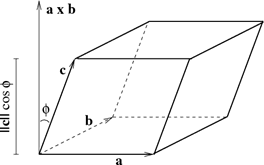# The Scalar Triple Product

The volume of a triangular prism is given by:

v = ½ |ab x c|

Where b and c are two of the sides of the triangular face of the prism, and a is the length of the prism.

The volume of a rectangular/parallelogram-based pyramid is given by:

V = ⅓ |a • b x c|

My question is, what are a, b, and c?
Is it necessary, in general, that b and c be the lengths of two of the sides of the base?

jedishrfu
Mentor
they are vectors that are the edges of a parallelogram when placed with tails coming from the same vertex.•PFuser1232
Well this topic regenerated the doubt that was in my mind some time ago.
Volume of parallelopiped is
[a b c]
Then how Volume of tetrahedron is
1/6 [a b c]

So the permutations of vectors in the triple product doesn't really matter, provided the absolute value is taken.
What matters is that they should be three vectors meeting at any vertex. Correct?

jedishrfu
Mentor
Yes and that defines the parallel piped too.